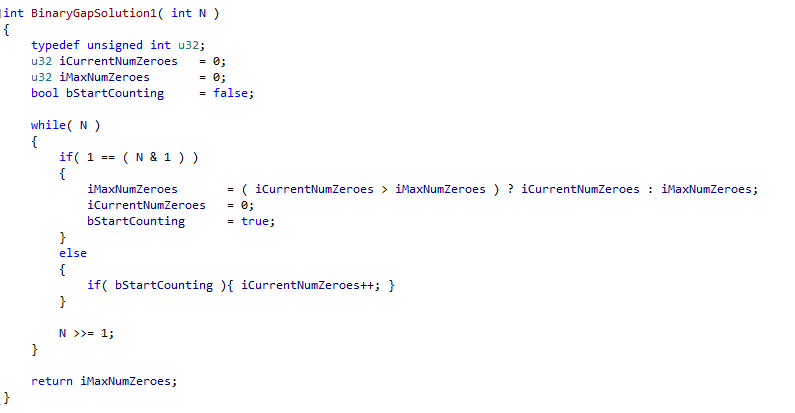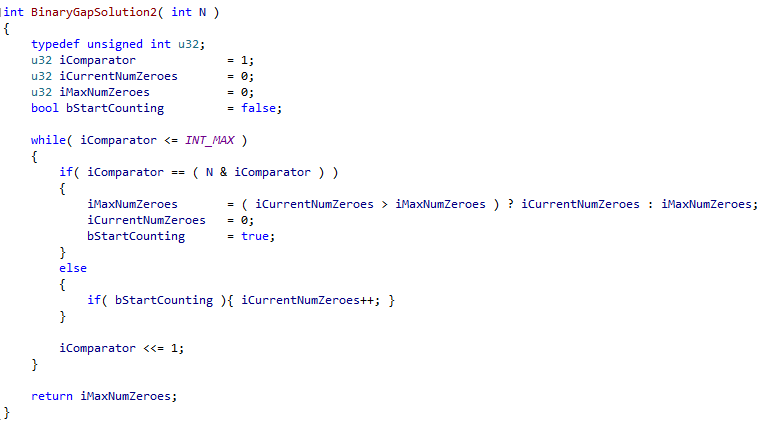### Binary Gap

You are to count the number of bits equal to zero in an integer between two bits equal to one. For example The number 529 has binary representation 1000010001 and contains two binary gaps: one of length 4 and one of length 3.

Therefore zeroes after the most significant ‘1’ will not be counted. Any number without a separated ‘string’ of zeroes, such as any power of two, should not have any gaps.

Assumptions:

N is an integer within the range [1..2,147,483,647].

The worst-case time complexity should be O(log(N)) .

Programming language used: C/C++

It helps to understand binary representation and the bitwise operator & for my solutions.

#### Solution 1 – shift downward

Shifting 1000010001 down by one bit will equal 0100001000. 1000010001 & 0000000001 is equal to 0000000001 or 1 in decimal. Therefore you exploit this to get your gap ‘ends’ and then count the zeroes inbetween. This will of course alter N, so you may want to use a temporary variable. We use set a boolean to true after our first ‘1’ to skip trailing zeroes.Solution 2 – shift upward

Similarly, rather than shifting down N we shift upward to it with a temporary variable to get our single ‘1’ bits. In my example below I shift up to 2,147,483,647, the maximum value for a signed integer, which we know is the maximum value for N, as this then tests my assumptions. Indeed, when N is this large you must use an unsigned counter, otherwise your temp var will always < 2,147,483,647 and you get an infinite loop:Solution 3- shift up through all powers of two

Similarly to above we can move through all powers of two up to the maximum for our integer  (which on my machine is 4 bytes x 8 bits = 32 bits) to get our ‘1’s, so that we do not need to bit shift a number at all. The bitwise AND of this number and our input number will indicate the presence of our ‘1’s.